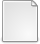# Nonlinear state feedback control for a class of polynomial nonlinear discrete-time systems with norm-bounded uncertainties: An integrator approach

Md Saat, Mohd Shakir (2013) Nonlinear state feedback control for a class of polynomial nonlinear discrete-time systems with norm-bounded uncertainties: An integrator approach. Journal of The Franklin Institute. pp. 1739-1752. ISSN 0016-0032Other (URL) JoFranklinInstituteApril2013.pdf - Published Version Restricted to Registered users only Download (397kB) | Request a copy

## Abstract

This paper investigates the problem of designing a nonlinear state feedback controller for a class of uncertain polynomial nonlinear discrete-time systems with norm-bounded uncertainties. In general, the problem of stabilising nonlinear discrete-time systems cannot be formulated as a convex problem. This is due to the fact that the Lyapunov function and the control input are not jointly convex, hence it cannot be solved by a semidefinite programming (SDP). In this paper, we propose a novel approach where an integrator is introduced to convexify this nonconvex controller design problem. Furthermore, based on the sum of squares approach, sufficient conditions for the existence of a polynomial nonlinear state feedback controller for polynomial nonlinear discrete-time systems are given in terms of solvability of polynomial matrix inequalities. These inequalities are then solved by the sum of squares (SOS) solvers. Finally, numerical examples are provided to demonstrate the validity of this integrator approach.

Item Type: Article T Technology > TK Electrical engineering. Electronics Nuclear engineering Faculty of Electronics and Computer Engineering > Department of Industrial Electronics DR. Mohd Shakir Md Saat 31 Jul 2013 04:21 28 May 2015 04:00 http://eprints.utem.edu.my/id/eprint/8999 View Download StatisticView Item